These Pages include various standards. To confirm the status of any standard, identify the replacement standard if it is obsolete and/or purchase the standard please use. BSI Shop It is also possible to become a BSI member and obtain copies of the Standards at much reduced prices.

Screws Index Page

Bolted Joint Design

Introduction

A most important factor is machine design, and structural design is the rigid fastening together of different components..This should include the following considerations..

 Assembly Accuracy of positioning Ability to Hold components rigidly together against all forces Requirement to separate components Retention of fastening over time

There are many methods of fastening items together including

 Bolting Rivetting Pins Keys Welding/Soldering/Brazing Bonding Velcro Magnetism

These notes relate primarily to the bolted joint.   The bolted joint is a very popular method of fastening components together.  The prime reason for selecting bolts as opposed to welding, or rivets is that the connection can be easily released allowing disassembly, maintenance and/or inspection..

The bolts /screws are generally used in groups to fasten plates together.   A bolt is a screwed fastener with a head, designed to be used with a nut.   A screw is a fastener designed to be used with a formed female thread in one of the components being attached.

These notes generally relate to bolts and nuts and hex headed screws..

A bolt can be loaded in one of three ways

 Tension Shear Combined Shear and Tension

Note: Conditions where bending loads are imposed on the bolt e.g. non-parallel bolting surfaces, should be avoided.

A bolt is primarily designed to withstand tensile loading while clamping components together.   Ideally the bolt should only be loaded in tension.  Any forces tending to slide the clamped components laterally should be withstood by separate means..

Holes for bolts are generally clearance holes and the best design of bolt is one with a reduced shank diameter (waisted shanks).   Joints in shear depending on the bolts to withstand the shear load are not really rigid.   Significant relative sideways movement must take place before the bolt shank can take any shear load (hole clearance).  It is also likely that in the case of components attached by a number of bolts that one bolt would be loaded first and this bolt would have to yield before the other bolts take their share of the shear load....

Bolts taking significant tensile and shear load need to be engineered to withstand the combined stress..

In structural engineering the codes identify the use of High Strength Friction Grip Bolts (Ref BS 4604 Pts 1-2:1970).   The bolts are tightened to a specified minimum shank tension so that transverse loads are transferred across the joint by friction between the plates rather than by shear across the bolt shank.

In mechanical engineering / machine engineering, items are often accurately located using dowels /locating pins.   When installed these dowels /locating pins should be engineered to withstand any traverse loads.   A recent innovation is to provide dowel bushings.  These are used in conjunction with bolts which pass through the inside of the bushing after it has been installed.   Separate holes for locating pins are eliminated.    The hardened bushings absorb shear loads, isolating the bolts from these forces.

If the choice is made that bolts/screws are to take shear load the joint should be arranged that the threaded portion of the bolt/screw shank is not taking the shear.

 The notes on this page relate to the mechanical engineering industry.. In the aerospace industry joints are often designed to specifically load the bolts in shear.   The screws and bolts used are high specification close toleranced items and the holes are also machined to close tolerances.  The bolted lap joints are generally used for critical assemblies and joints designed with bolts loaded in tension are avoided.????

Strength of Bolts in Shear

Important Note:
The calculations below are based on the unrealistic assumption that there is no friction forces between the plates which are clamped by the bolts.  The calculations are therefore conservative (safe)..

For bolts joints loaded in shear - three stress areas result-

 The bolts are loaded in shear..Depending on the joint design the bolt can be in single or double shear... The bolt interface with the hole is compressively loaded. ( Crushing ) If the hole is near to the edge of the plate the plate is subject to shear loading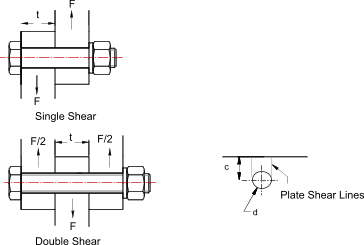Single Shear..

Shear Stress = 4 . F / π. d 2
Compressive Stress = F / (d . t)
Plate Shear Stress = F / (2.c.t)

Double Shear ..

Shear Stress = 2 . F / π. d 2
Compressive Stress = F / (d . t)
Plate Shear Stress = F / (2.c.t)

The stresses are adjusted based on the number of bolts / screws used for the joint..

Consider a bracket taking an offset load F (N) at a radius R (m).  The bracket is secure using a number of bolts each with a Area A(m2 ).   The bolts are located around a centroid position each with a radius from the centroid of rn(m) and a horizontal/vertical position relative to the centroid of hn /vn (m) . ( bolt is designated by the subscript "n". )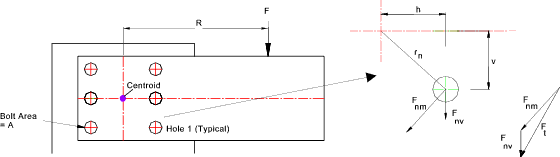Location of Centroid... The location of the centroid of the bolts can often be determined by inspection as in figure above.   If the bolts are not arranged around a convenient centre then the centroid is determined by .. x position = sum of the moments of area of all the holes about a fixed horizontal position divided by the total hole area y position = sum of the moments of area of all the holes about a fixed vertical position divided by the total hole area

The offset load is equivalent to a vertical force (F) + moment (F. R) at the centroid of the bolts...

Each bolt is withstands a vertical shear force      Fnv = F / No of Bolts.
Each bolt also withstands a shear load      Fnm = F.R. rn / (r12 + r22...rn2)
The total horizontal force on each bolt      Fth= Fnm . vn / Sqrt(hn2 + vn2 )
The total vertical force on each bolt     Ftv= Fnv + Fnm . hn / Sqrt(hn2 + vn2 )
The total shear load on each bolt     Ft= Sqrt (Fth2 + Ftv2)
The resulting bolt shear stress     τ t = Ft /A

The shear stress in each bolt is calculated to ensure the design is safe..

Strength of bolt joints withstanding bending forces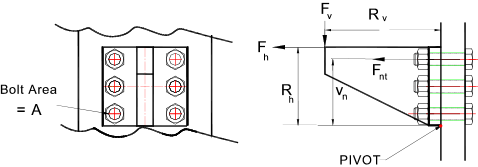Each Bolt withstands a shear Force     Fs = Fv / (Number of bolts)
The resulting shear bolt stress     τ n = Fs /A

Note: Each bolt is assumed to withstand the same shear force.

If there are x bolts( numbered n = 1 to x). Then the tensile force withstood be each bolt is designated Fnt i.e F1t,F2t, F3t....Fxt
A selected bolt (n) withstands a tensile force of     Fnt = ( Fv. Rv + Fh. Rh) . Vn / (V12 + V22....Vx2 )
The resulting tensile bolt stress     sn = Fnt /A

Maximum principal tensile stress in the bolt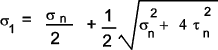Maximum principal compressive stress in the bolt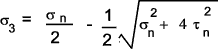Maximum shear stress in the bolt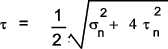Failure criteria: Refer to page Failure Modes

The notes on this page In order to estimate the design factors of safety it is necessary to consider the failure modes.    The preferred failure criteria for ductile metals is the "Shear Strain Energy Theory" (Von Mises-Hencky theory).   For a stress regime associated with a bolt i.e pure tensile stress sx combined with shear stress τ xy.   The Factor of safety relative to the material tensile strength Sy..is calculated as follows

Factor of Safety = Sy / ( sx2 + 3 .τ xy2 ) 1/2